## Ex 4.4 Question 1.

1. Draw a rough sketch of a triangle ABC. Mark a point P in its interior and a point Q in its exterior. Is the point A in its exterior or in its interior?

Solution: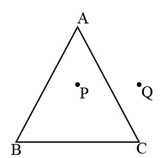Point A is neither interior nor exterior of the triangle. It is a vertex.

## Ex 4.4 Question 2.

2. (a) Identify three triangles in the figure.

(b) Write the names of seven angles.

(c) Write the names of six line segments

(d) Which two triangles have ∠B as common?Solution:

(a) The three triangles = ∠ABD, ∠ACB, ∠ADC

(c) The line segments =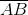,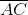,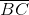,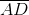,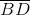,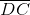(d) ∠ABD and ∠ABC are triangles that have ∠B as common.

error: Content is protected !!